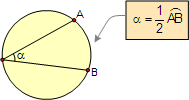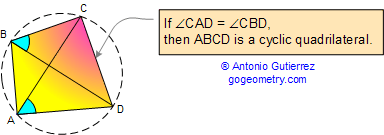# Geometry Problem 77. Angles in a circle, Cyclic Quadrilateral, Parallel lines. Level: College, High School.

 Given a circle (1), the lines AED (2) and BFC (3). If AB is parallel to CD, prove that the angles AFB and AEB are equal. View or post a solution.HINTS:

PARALLEL LINES

Proposition. If two lines are parallel, each pair of alternate interior angles are congruent. Also converse.ANGLES IN A CIRCLE

Proposition.  An inscribed angle is measured by one-half its intercepted arc.CYCLIC QUADRILATERAL is a quadrilateral whose vertices all lie on a single circle.

Proposition 1. Opposite angles of a cyclic (inscribed) quadrilateral are supplementary. Also converse.Proposition 2. A quadrilateral is cyclic if one side subtends congruent angles at the two opposite vertices. Also converse.